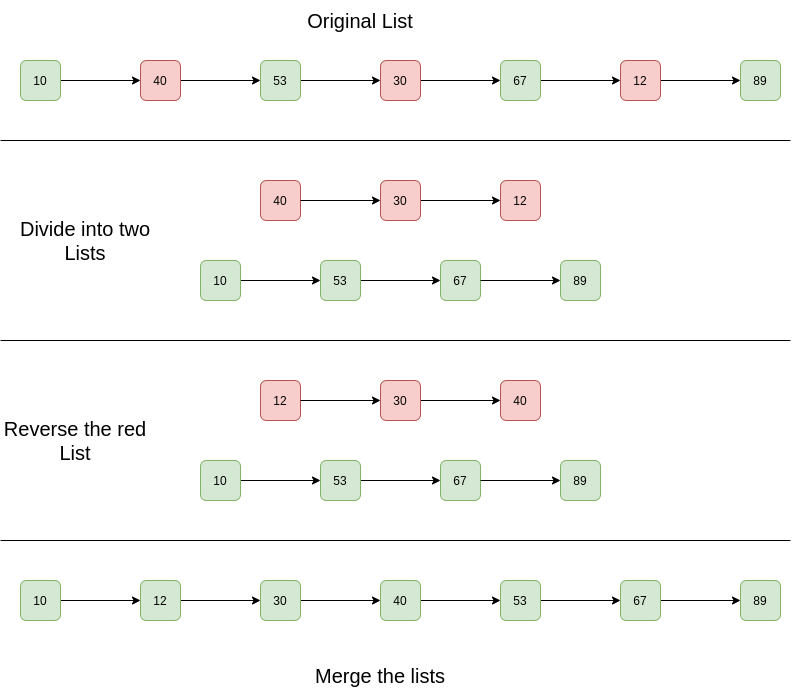Open In App

# Javascript Program For Sorting A Linked List That Is Sorted Alternating Ascending And Descending Orders

Given a Linked List. The Linked List is in alternating ascending and descending orders. Sort the list efficiently.

Example:

```Input List: 10 -> 40 -> 53 -> 30 -> 67 -> 12 -> 89 -> NULL
Output List: 10 -> 12 -> 30 -> 40 -> 53 -> 67 -> 89 -> NULL

Input List: 1 -> 4 -> 3 -> 2 -> 5 -> NULL
Output List: 1 -> 2 -> 3 -> 4 -> 5 -> NULL```

Simple Solution:

Approach: The basic idea is to apply to merge sort on the linked list.

Complexity Analysis:

• Time Complexity: The merge sort of linked list takes O(n log n) time. In the merge sort tree, the height is log n. Sorting each level will take O(n) time. So time complexity is O(n log n).
• Auxiliary Space: O(n log n), In the merge sort tree the height is log n. Storing each level will take O(n) space. So space complexity is O(n log n).

Efficient Solution:
Approach:

1. Separate two lists.
2. Reverse the one with descending order
3. Merge both lists.

Diagram:Below are the implementations of the above algorithm:

## Javascript

 ``

Output:

```Given Linked List is
10 40 53 30 67 12 89